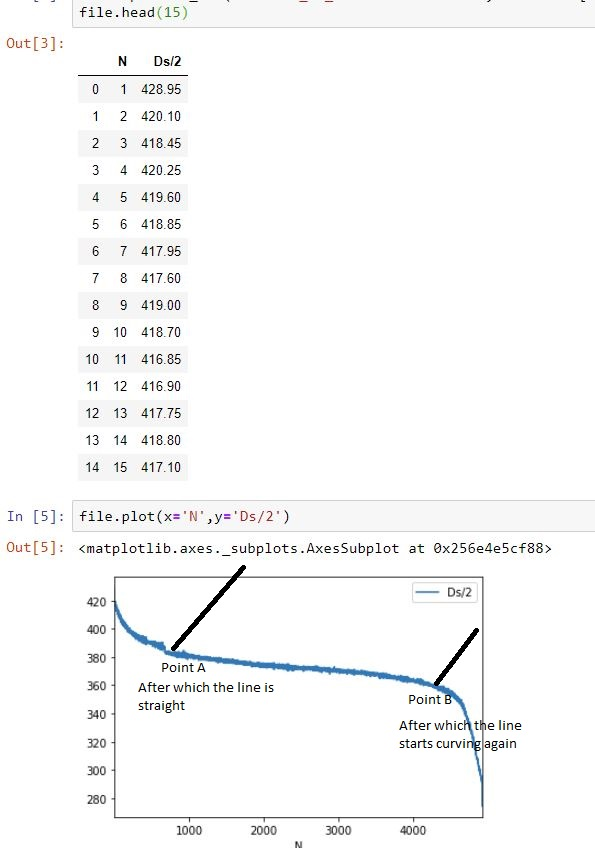关于python：在曲线上找到两个点/导数，其间的直线/常数

2022-01-08

Find two points/derivatives on curves between which the line is straight/constant1234567891011 import numpy as np import matplotlib.pyplot as plt from scipy.interpolate import UnivariateSpline x = file['n'] y = file['Ds/2'] y_spline = UnivariateSpline(x, y) x_range = np.linspace(x, x[-1], 1000)  # or could use x_range = x y_spline_deriv = y_spl.derivative(n=2) curvature = y_spline_deriv(x_range)

 12345 straight_points = np.where(curvature.abs() <= 0.1)  # pick your threshold start_idx = straight_points end_idx = straight_points[-1] start_x = x_range[start_idx] end_x = x_range[end_idx]